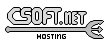<-- Back to AG_Intro.3

# SYNOPSIS

 ```#include #include #include ```

# DESCRIPTION

The M_Triangle2 structure describes a triangle in R2 in terms of three lines:
 ```typedef struct m_triangle2 { M_Line2 a, b, c; } M_Triangle2; ```

Similarly, M_Triangle3 describes a triangle in R3:
 ```typedef struct m_triangle3 { M_Line3 a, b, c; } M_Triangle3; ```

# INITIALIZATION

 M_Triangle2 M_TriangleFromLines2 (M_Line2 L1, M_Line2 L2, M_Line2 L3)M_Triangle3 M_TriangleFromLines3 (M_Line3 L1, M_Line3 L2, M_Line3 L3)M_Triangle2 M_TriangleFromPts2 (M_Vector2 a, M_Vector2 b, M_Vector2 c)M_Triangle3 M_TriangleFromPts3 (M_Vector3 a, M_Vector3 b, M_Vector3 c)M_Triangle2 M_TriangleRead2 (AG_DataSource *ds)M_Triangle3 M_TriangleRead3 (AG_DataSource *ds)void M_TriangleWrite2 (AG_DataSource *ds, M_Triangle2 *T)void M_TriangleWrite3 (AG_DataSource *ds, M_Triangle3 *T)M_Triangle2 M_TRIANGLE2_INITIALIZER (M_Vector2 a, M_Vector2 b, M_Vector2 c)M_Triangle3 M_TRIANGLE3_INITIALIZER (M_Vector3 a, M_Vector3 b, M_Vector3 c)

The functions M_TriangleFromLines2() and M_TriangleFromLines3() return an M_Triangle2 or M_Triangle3 describing a triangle in terms of three lines L1, L2 and L3. The three lines must be non-colinear and their endpoints must coincide.

M_TriangleFromPts2() and M_TriangleFromPts3() return a triangle in terms of three (non-colinear) points a, b, c.

The M_TriangleRead() and M_TriangleWrite() functions read or write a triangle structure from/to an AG_DataSource(3).

The macros M_TRIANGLE2_INITIALIZER() and M_TRIANGLE3_INITIALIZER() expand to static initializers for M_Triangle2 and M_Triangle3, respectively.

# COMPUTATIONS

 int M_PointInTriangle2 (M_Triangle2 T, M_Vector2 p)

The M_PointInTriangle2() routine tests (using barycentric coordinates) whether point p lies within the triangle T and returns 1 if it does, otherwise 0.